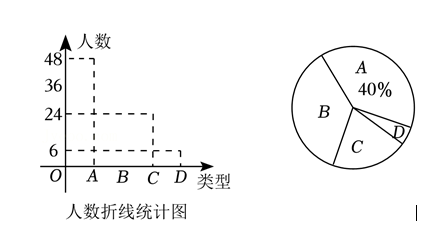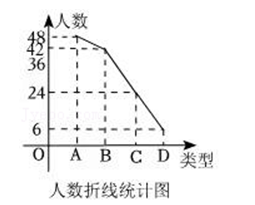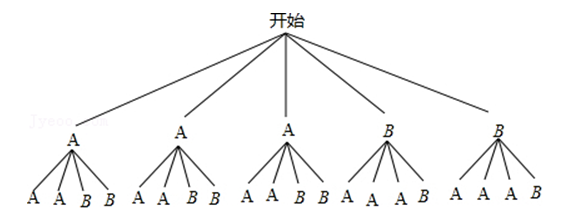(1) 扇形统计图中 $D$ 类所对应的圆心角度数为 , 并补全折线统计图;
(2) 现从 $A$ 类中选出两名男同学和三名女同学, 从以上五名同学中随机抽取两名同学进 行采访, 请利用画树状图或列表的方法求出抽到的学生恰好是一男一女的概率.【解答】解: (1) $\because$ 被调查的总人数为 $48 \div 40 \%=120$ (人),
$\therefore$ 扇形统计图中 $D$ 类所对应的圆心角度数为 $360^{\circ} \times \frac{6}{120}=18^{\circ}$,
$B$ 类型人数为 $120-(48+24+6)=42$ (人),（2）A表示女生，B表示男生①点击 首页查看更多试卷和试题 , 点击查看 本题所在试卷